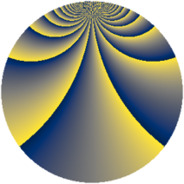# Properties

 Label 2760.2.brLevel $2760$ Weight $2$ Character orbit 2760.br Rep. character $\chi_{2760}(1333,\cdot)$ Character field $\Q(\zeta_{4})$ Dimension $576$ Sturm bound $1152$

# Related objects

## Defining parameters

 Level: $$N$$ $$=$$ $$2760 = 2^{3} \cdot 3 \cdot 5 \cdot 23$$ Weight: $$k$$ $$=$$ $$2$$ Character orbit: $$[\chi]$$ $$=$$ 2760.br (of order $$4$$ and degree $$2$$) Character conductor: $$\operatorname{cond}(\chi)$$ $$=$$ $$920$$ Character field: $$\Q(i)$$ Sturm bound: $$1152$$

## Dimensions

The following table gives the dimensions of various subspaces of $$M_{2}(2760, [\chi])$$.

Total New Old
Modular forms 1168 576 592
Cusp forms 1136 576 560
Eisenstein series 32 0 32

## Trace form

 $$576q + 8q^{6} + O(q^{10})$$ $$576q + 8q^{6} - 8q^{16} - 32q^{31} - 40q^{32} + 8q^{36} + 48q^{46} - 32q^{48} + 32q^{50} + 112q^{52} - 32q^{58} + 112q^{62} + 32q^{70} - 576q^{81} - 40q^{82} - 24q^{92} - 88q^{96} + 56q^{98} + O(q^{100})$$

## Decomposition of $$S_{2}^{\mathrm{new}}(2760, [\chi])$$ into newform subspaces

The newforms in this space have not yet been added to the LMFDB.

## Decomposition of $$S_{2}^{\mathrm{old}}(2760, [\chi])$$ into lower level spaces

$$S_{2}^{\mathrm{old}}(2760, [\chi]) \cong$$ $$S_{2}^{\mathrm{new}}(920, [\chi])$$$$^{\oplus 2}$$